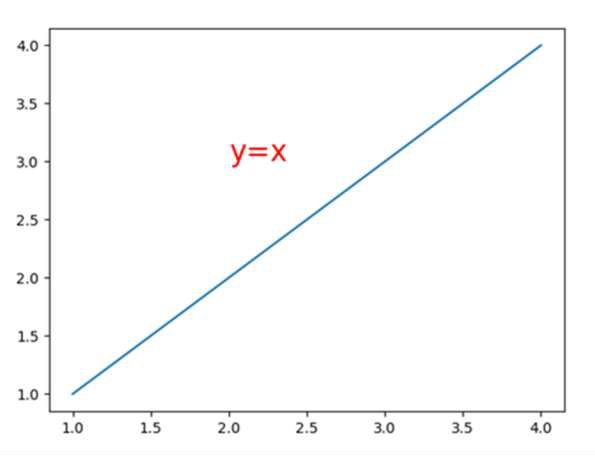# How to change fonts in matplotlib (python)?

Using plt.text() method, we can increase the font size.

## Steps

• Using plt.plot() method, we can create a line with two lists that are passed in its argument.

• Add text to the axes. Add the text *s* to the axes at location *x*, *y* in data coordinates, using plt.text() method. Font size can be customized by changing the font-size value.

• To show the figure, use plt.show() method.

## Example

import matplotlib.pyplot as plt

plt.plot([1, 2, 4], [1, 2, 4])

plt.text(2, 3, "y=x", color='red', fontsize=20)
# Increase fontsize by increasing value.

plt.show()

## OutputUpdated on: 15-Mar-2021

2K+ Views